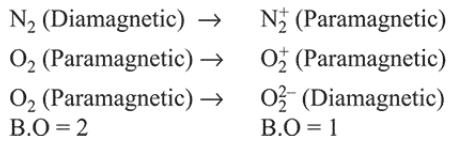# In which of the following processes,

Question:

In which of the following processes, the bond order has increased and paramagnetic character has changed to diamagnetic?

1. $\mathrm{NO} \rightarrow \mathrm{NO}^{+}$

2. $\mathrm{N}_{2} \rightarrow \mathrm{N}_{2}^{+}$

3. $\mathrm{O}_{2} \rightarrow \mathrm{O}_{2}^{+}$

4. $\mathrm{O}_{2} \rightarrow \mathrm{O}_{2}^{2-}$

Correct Option:

Solution:

In case of $\mathrm{NO}$ (paramagnetic) $\rightarrow \mathrm{NO}^{+}$(diamagnetic) the bond order has increased from $2.5$ to 3 .

For other cases: